﻿ Fuzzy Logic and PID Controllers for DC Motor Using Genetic AlgorithmInternational Journal of Control Science and Engineering

p-ISSN: 2168-4952    e-ISSN: 2168-4960

2020;  10(2): 37-41

doi:10.5923/j.control.20201002.03

Received: Dec. 3, 2020; Accepted: Dec. 19, 2020; Published: Dec. 26, 2020### Fuzzy Logic and PID Controllers for DC Motor Using Genetic Algorithm

Md. Turiqul Islam, S. M. Rezaul Karim, Avizit Sutradhar, Shahin Miah

Department of EEE, International University of Business Agriculture and Technology, Dhaka, Bangladesh

Correspondence to: Md. Turiqul Islam, Department of EEE, International University of Business Agriculture and Technology, Dhaka, Bangladesh.
 Email: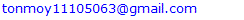Abstract

In this article, DC motor supply is controlled using PID controller and fuzzy logic controller. The PID controller requires a mathematical model of the system, while the obscure logic controller is based on experience with rule-based information. The design of a fuzzy logic controller requires a number of design decisions, such as rule-based and physics. FLC has two inputs, one of which is a speed error and the other is a change in speed error. Here are 49 Fuzzy rules designed for the controller of Fuzzy logic. The center of gravity method is used for defasification. The Fuzzy logic controller has used a civilized system that results in Fiji set employees. The PID controller selects its parameters based on trial and error. Method PID and FLC are explore with the help of MATLAB / SIMULINK package program simulation. It has been established that the FLC is more difficult in design to compare with the PID controller, but it has to be more suitable to meet the non-linear characteristics of the DC motor. The results show that fuzzy logic has minimal transient and stable state parameters, indicating that the FLC & PID is more efficient and effective than the controller.

Keywords: Genetic Algorithm, Fuzzy system, Machine learning, Applying Genetic Algorithm, Mat Lab work

Cite this paper: Md. Turiqul Islam, S. M. Rezaul Karim, Avizit Sutradhar, Shahin Miah, Fuzzy Logic and PID Controllers for DC Motor Using Genetic Algorithm, International Journal of Control Science and Engineering, Vol. 10 No. 2, 2020, pp. 37-41. doi: 10.5923/j.control.20201002.03.

### 1. Introduction

Almost each mechanical movement that has been detected around United States of America is accomplished by an electrical motor. Electrical machines square measure a way of switching energy. Motors take electricity and manufacture energy. Electrical motors square measure accustomed power many devices we have a tendency to use in lifestyle. Electric motors square measure generally classified into 2 totally different categories: DC (Direct Current) and AC (Alternating Current). Inside these classes square measure various sorts, every giving distinctive talents that suit them well for specific applications. In most cases, in spite of sort, electrical motors carries with it a mechanical device (stationary field) and a rotor (the rotating field or armature) and operate through the interaction of magnetic flux and electrical phenomenon to supply motility speed and torque" .

### 2. Fuzzy -PID Controller

The term PID is derived from proportional component der and is a type of instrument used to control various process variables such as pressure, flow, temperature and speed of industrial applications. In this controller, a control loop feedback tool is used to manage the process variables.
Such controls are used to operate the system in the direction of a target location, otherwise on the surface. It is present almost everywhere for temperature control and is used in scientific processes, automation and various chemicals. In this controller, the use of closed loop feedback is used to maintain the actual output in some way, such as being close to the object, otherwise the output at a fixed point if possible. In this article, PID controller designs that use P, I&D as well as control mode are discussed [2,3].
Due to the high speed management options of DC motors, they're wide utilized in business for varied applications specifically AI, rolling mills, machine tools, position management, mining, paper and textile producing etc. There are. PID management is employed as a regular technique. For the aim of dominant DC motors. However, it cannot effectively deal with load variations and changes in model parameters. To beat these limitations, over the past 20 years, many new management techniques to manage DC motor speeds, like neural networks, genetic algorithms, and mathematical logic are combined with classic PID.

#### 2.1. Fuzzy Inference System

The Fuzzy Infinity System (FIS) is the process of generating an input-to-output mapping using a Fuzzy logic. Automatic control, data classification, decision analysis, expert systems, and computer Fuzzy infinity systems have been used successfully in areas such as vision .
A singleton, or more precisely an ambiguous singleton, is a fuzzy set that has a membership function that is unity at a particular point in the universe and zero anywhere. "The need to use FLC arises fundamentally in situations where:
• The description of a technical process is only available in verbal form, not in analytical form.
• The process parameters cannot be accurately identified.
• The explanation of the process is very complex and it is more reasonable to explain it in simple words.
• Technical has a "fuzzy" role in controlled technical processes.
• It is not possible to explain these situations clearly.

#### 2.2. Fuzzy Controller

Fuzzy Logic Control is a control algorithm based on a language control strategy, taken from an expert in an automated control strategy. Fuzzy 3-1 provides a block diagram for the Fuzzy control system. The Fuzzy Controller consists of the following four components .
• Fuzzification
• Rule Base
• Inference Engine
• De-fuzzification
The details of each chapter are given further in this chapter.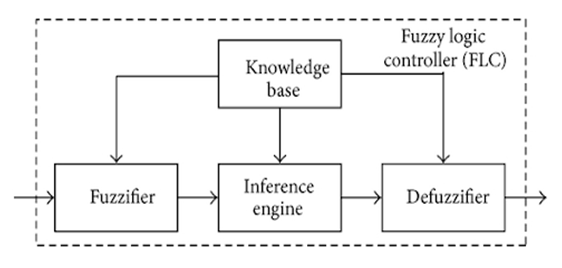Figure 1. Fuzzy Logic Controller

#### 2.3. Fuzzification

The first step in plotting an obscure controller is to decide which state represents the dynamic performance of the variable system. The controller should be taken as the input signal. Ambiguous logic uses linguistic variables instead of numerical variables . The process of converting a numerical variable (real number or crisp variable) into a linguistic variable (fuzzy number) is called physics. It is obtained with different types of fuzzifiers. There are basically three types of fuzzifiers, which are used for the fuzzification process. They are
• Singleton fuzzifier
• Gaussian fuzzifier
• Trapezoidal or triangular fuzzifier

#### 2.4. Rule Base

A decision-making logic that mimics the human decision-making process eliminates the ambiguous control process with knowledge of control rules and linguistic variable definitions. The rules are in the "if then" format and formally if the party is called the terms and then the side is called the closing . A control based on the application of computer rules and measuring input error (e) "difference between output speed and set point" and the variability of the error depending on the output of the fuzzy controller and control function. Able to count signals. The armature will be the voltage. In a rule base controller the control approach is stored in a more or less natural language. A rule base controller is easy to understand and easy to maintain for a non-specialist end user and an equivalent controller could be implemented using conventional techniques . The rules are illustrated in table 2 (7 * 7 = 49).
• LN: Large Negative
• MN: Medium Negative
• SN: Small Negative
• ZE: Zero
• SP: Small Positive
• MP: Medium Positive

#### 2.5. De- Fuzzification

The reversal of fusion is called deification. Using the Fuzzy Logic Controller (FLC) generates the desired output in the linguistic variable (Fuzzy number). Linguistic variables have to be converted to crisp production according to real world needs . There are many differentiation methods but the most common is:
Center of Gravity (COG): For single sets, the COG is called the center of gravity for Singleton (COGS) where the center of gravity of the crisp control value is summarized as follows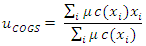Where XI is a point in the end universe (i = 1, 2, 3…) and (c (XI) is the value of the membership of the end set of the result.

### 3. Literatures Review

This work deals with the appliance of organic process computation in approximation and management of linear time invariant (LTI) systems. Random shape search formula (SFS) has been planned to get low order system (LOS) from LTI higher order system (HOS) likewise as in speed management of DC motor with inflammatory disease controller. SFS is sort of straightforward to use up to speed system and employs the diffusion property gift in random fractals to discover the search house. In approximation of LTI systems, the integral sq. error (ISE) whereas up to speed of DC motor, the integral of your time increased absolute error has been taken as associate degree objective/fitness operate.. The simulation results have additionally been compared in terms of; ISE integral absolute error and impulse response energy with well-known acquainted and recently revealed works within the literature that shows the prevalence of SFS formula. Up to speed of DC motor, the obtained results are satisfactory having no overshoot and fewer rise and obtained results are satisfactory having no overshoot and fewer rise.

### 4. Methodology

It is a well-known fact that the performance of the Fuzzy PID controller depends on the correct value of its parameters Kp, Ki and Kd. There are many ways to find the maximum value of these parameters so that the plant production remains in the satisfied area. Of the many methods, the most commonly used and powerful method is the one used to suit the parameters of any controller. Figure 2 shows the optimization procedure to conform to the parameters of the basic controller.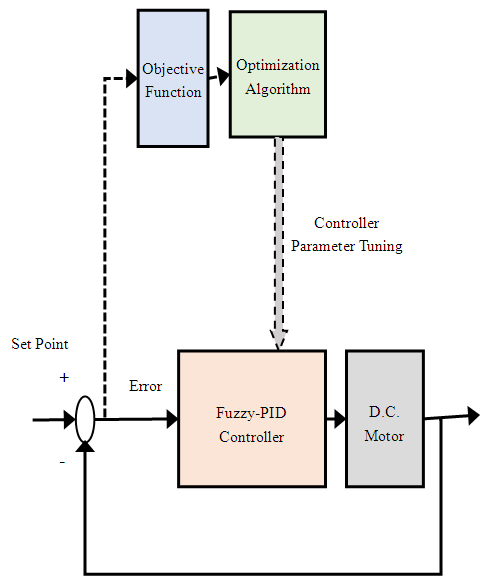Figure 2. Block Diagram of Optimization Procedure

#### 4.1. Objective Function

It is clear that the objective function plays an important role in finding the best possible value of controller parameters. The optimization algorithm seeks to minimize the work of purpose by generating different values for Kp, Ki and Kd. In many literatures, objective function is also called cost work.
The object is a mathematical equation that depends on the value of the error. There are basically four types of objective functions used in control system disturbance. These reasonable functions are known as Integral Absolute Error (IAE), Integral Square Error (ISE), Integral Time Absolute Error (ITAE) and Integral Time Square Error (ITSE). The mathematical equations of the four objective functions are given by the unit. (1) - Eq. (4)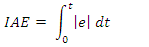(1)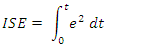(2)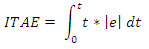(3)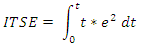(4)
The performance of the optimization algorithm depends very much on the type of purpose work. There are many research papers in the control system literature that have evaluated the performance of optimization algorithms for different purpose functions. The general conclusion is that objective functions based on ITAE perform better than other objective functions. That is why in this work we have also used ITAE as the objective function of our optimization algorithms.

#### 4.2. Optimization Algorithm

Genetic algorithm (GA) optimization modeling is used to find the parameters of the fuzzy-PID controller for a DC motor. The following rules have been adopted:
Data creation: A random sequence of populations occurs when the initial population controller configures the parameters 𝐾𝑝, 𝐾𝑖, 𝐾𝑑, x and y by encoding the names of the binary strings of the chromosome [10,11].
Accuracy and approximation: The fitness function is obtained to convert the binary values of the strings created (part 1) into real values representing the controller parameters. Cost Function Correction: The original values (found in Part 2) apply to the CFOPID parameters, the answer is viewed and the error is calculated. Cost work is determined based on the relevant fitness functions. Cost functions can range from system sensitivities to malfunction specifications such as ISE, IAE, etc. to achieve plant transfer function margins and phase margins with the entire controller.

### 5. Results & Discussion

The results of the optimization obtained from the algorithm (Genetic Algorithm) (GA). The production efficiency of the plant has also been tested in terms of plant production to refuse load disruption.
SIMULINK is used to create Simulink models for speed control of DC motors. The block diagram for ITA calculation is also shown in the figure. Figure 5-1 shows the diagram of the proposed model in Simulink. This model was used in the optimization process using genetic algorithms. Genetic algorithms were run for 50 iterations of population values 50.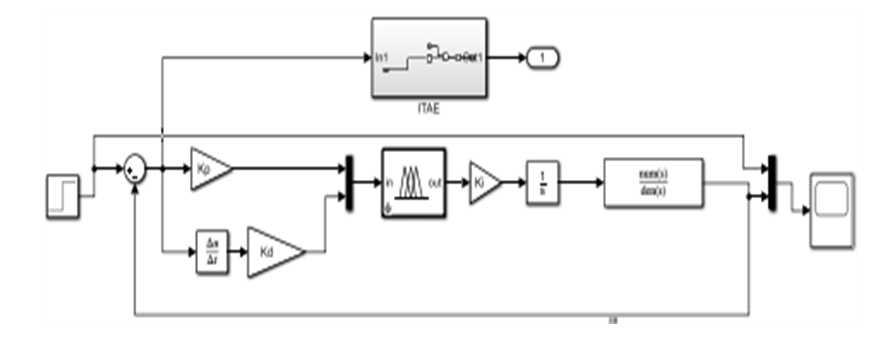Figure 3. Closed loop Simulink model for speed control of DC Motor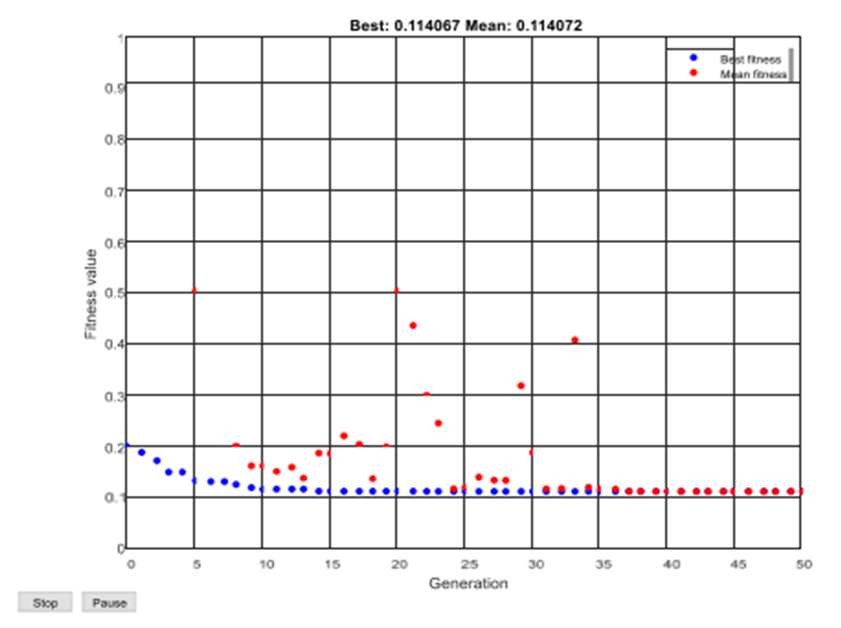Figure 4. Objective function value v/s no. of iterations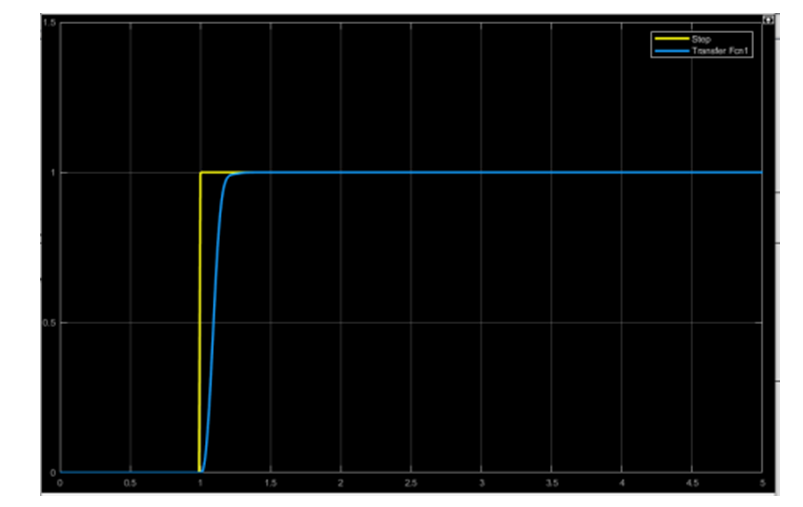Figure 5. Step response of the proposed Fuzzy-PID contro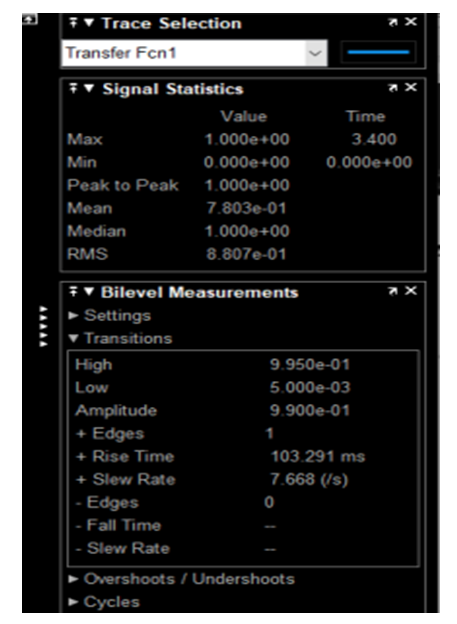Figure 6. Response Statistics of the Response under observation

### 6. Conclusions

In this report, the Fuzzy PID controller is designed and simulated to control the speed of a DC motor. The genetic optimization algorithm was used to adapt the parameters of the proposed Fuzzy-PID controller. Integrated Time Absolute Error (ITAE) was used as the purpose of the correction algorithm. It has been shown that the response of the tuned controller is much better than the open loop response of the system. Moreover, the system was found to be satisfactory. In addition, time extensions, scheduling, and overshoots were also used to account for the phased response of the calculation system.

### References

  M. Shadkam, H. Mojallali, Y. Bostani, “Speed control of DC motor using extended Kalman Filter Based fuzzy-pid”, International Journal of Information and Electronics Engineering, vol. 3, no. 1, January 2013.  Y.A. Almatheel, A. Abdelrahman, “Speed control of DC motor using fuzzy logic controller,” 2017 International conference on Communication, Control, Computing and Electronics Engineering (ICCCCEE), Khartoum, Sudan, 2017.  J. Agarwal, G. Parmar, R. Gupta, A. Sikander, “Analysis of grey wolf optimizer based fractional order PID controller in speed control of DC motor,” microsystem technologies, April 2018.  R. Bhatt, G. Parmar, R. Gupta, A. Sikander, “Application of stochastic fractal search in approximation and control of LTI systems”, Microsystem technologies, May 2018.  B. Hekimoglu, “Optimal tuning of fractional order PID controller for DC motor speed control via chaotic atom search optimization algorithm”, IEEE Access, 2019.  F. Rashidi, M. Rashidi, and A. H. Hosseini, “Speed regulation of DC motors using intelligent controllers,” in Proc. IEEE Conference on Control Applications, 2003, pp. 925 - 930.  K. K. Ahn and D. Q. Truong, “Online tuning fuzzy PID controller using robust extended Kalman filter,” Journal of Process Control, vol. 19, no.6, pp. 1011–1023, 2009.  S. Tiwari, A. Bhatt, A. C. Unni, J. G. Singh and W. Ongsakul, "Control of DC Motor Using Genetic Algorithm Based PID Controller," 2018 International Conference and Utility Exhibition on Green Energy for Sustainable Development (ICUE), Phuket, Thailand, 2018, pp. 1-6.  Thomas N, “Position control of DC motor using genetic algorithm based PID controller.” In: Proceeding of the World Congress on Engineering, WCE 2009, July 1–3, vol II. London, UK, pp 1618–1622, 200.  Y. Tipsuwan and Mo-Yuen Chow, "Fuzzy logic microcontroller implementation for DC motor speed control," IECON'99. Conference Proceedings. 25th Annual Conference of the IEEE Industrial Electronics Society (Cat. No. 99 CH37029), San Jose, CA, USA, 1999, pp. 1271-1276 vol.3.  S. P. Kumar, S. K. Veni, Y.B. Venugopal and Y. S. Kishore Babu, "A Neuro-Fuzzy based Speed Control of Separately Excited DC Motor", IEEE Transactions on Computational Intelligence and Communication Networks, pp. 93-98, 2010.